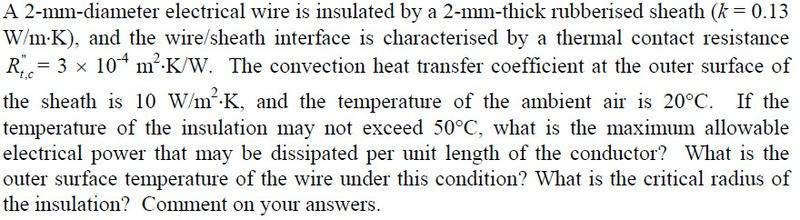# Heat Transfer - Heat Flux (thermal Resistance)

## Homework StatementHi guys,

I don't really need help with answering the dollowing question but just have a quick question about it.

I solved the first part of the question with an energy balance by letting $q_{conduction}$ = $q_{convection}$ to get the temperature of he outer surface temperature of the wire.

For the heat flux part, why can't the heat flux = $q_{conduction}$ = $q_{convection}$? Shouldn't the heat flux be constant throughout?

Chestermiller
Mentor

## Homework StatementHi guys,

I don't really need help with answering the dollowing question but just have a quick question about it.

I solved the first part of the question with an energy balance by letting $q_{conduction}$ = $q_{convection}$ to get the temperature of he outer surface temperature of the wire.

For the heat flux part, why can't the heat flux = $q_{conduction}$ = $q_{convection}$? Shouldn't the heat flux be constant throughout?